# Lions Weekly: August 10, 2022# A Note From Mr. Sandefur

Lion Family,

It is hard to imagine that we have completed our fifth day of instruction and are finishing our first full week of the academic year. It has been incredibly exciting having students back on campus as our halls are full of learning, laughter, and excitement. As we settle into a new year, it is the desire of our faculty and staff to partner with our families to impact our students for Christ and to grow them academically.

Please establish and develop a healthy communication line with your child’s teacher so they can assist you and your child with meeting their academic needs. We fully believe that through our partnership, students will continue to grow and develop into the young men and women that God has called them to be where they will be able to change the world through Christ.

In Christ alone,

Jeremy Sandefur

 table div table+table+table+table div table{width:100%;padding:0}table div table+table+table+table div table img{width:96.23%;padding:0;float:none}table div table+table+table+table div table td{width:100%;padding:0 1.88% 18px}/* styles */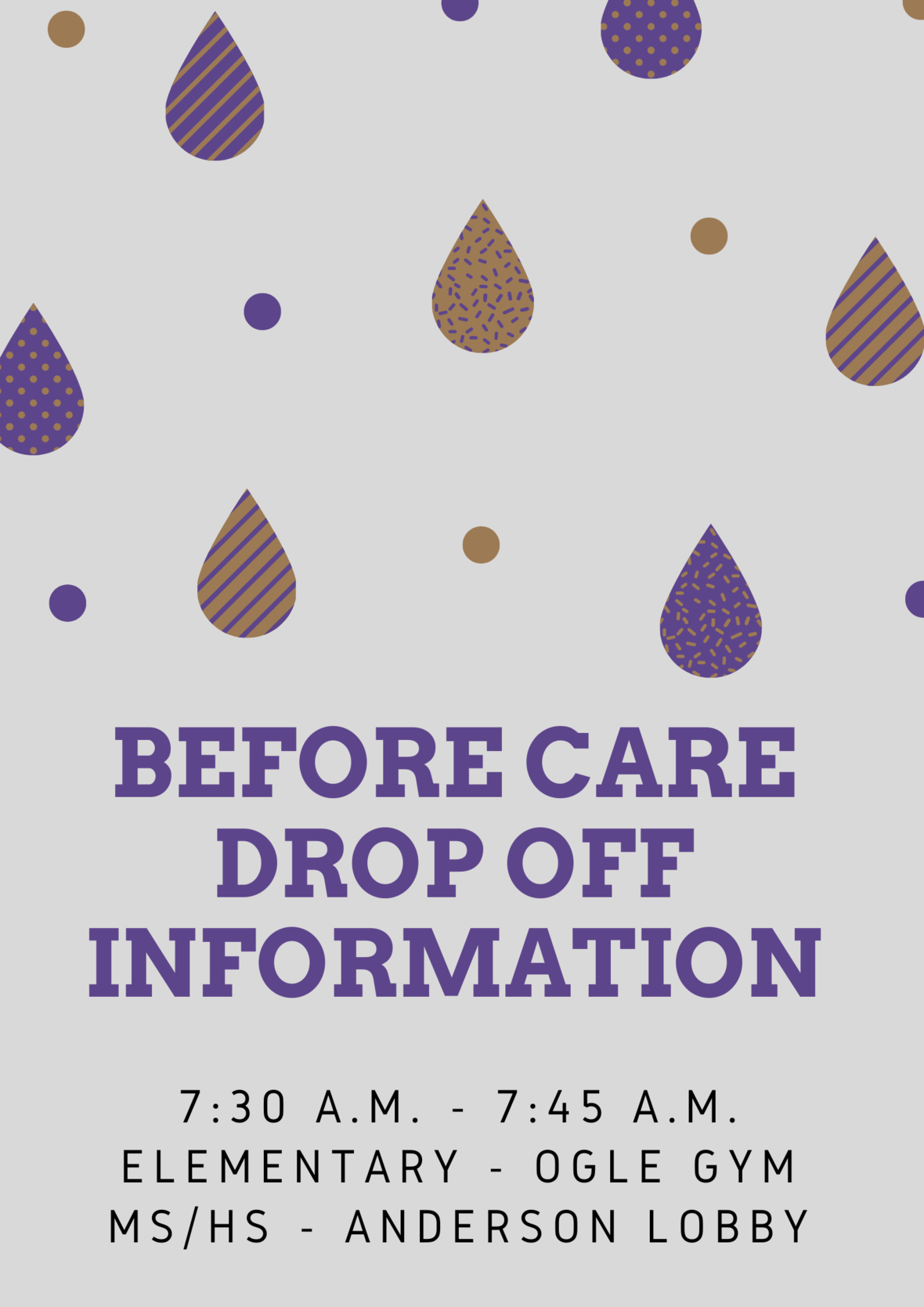# Before Care Information

Before care is provided from 7:30 a.m.- 7:45 a.m. on a daily basis for those who need it.

Classrooms/Locker Halls will open at 7:45 a.m. for students to be prepared for class to start at 8:00 a.m.

 table div table+table+table+table+table+table div table{width:100%;padding:0}table div table+table+table+table+table+table div table img{width:96.23%;padding:0;float:none}table div table+table+table+table+table+table div table td{width:100%;padding:0 1.88% 18px}/* styles */# PreK and Elementary Dismissal

Kindergarten through 5th grade dismissal carpool line begins at 2:45 PM. If you arrive prior to 2:40 PM, please park to allow the the PreK students to be dismissed. If you would like to park and pick up your child at the front of Ogle, please let your child know in advance. Thank you for helping our PreK and Elementary dismissals run smoothly.

Please have card tags visible and ready for pick up. If a teacher does not recognize the person, and they do not have a car tag, they will be asked to park and come into the front office to share their ID.

 table div table+table+table+table+table+table+table+table+table div table{width:100%;padding:0}table div table+table+table+table+table+table+table+table+table div table img{width:96.23%;padding:0;float:none}table div table+table+table+table+table+table+table+table+table div table td{width:100%;padding:0 1.88% 18px}/* styles */# Fall 2022 Dress Up Day Themes

Remember, all attire must adhere to dress code

▪ Friday, August 19 - Bright Out Spirit Day (Dress in Neon)
▪ Friday, August 26 - Surfing to Victory (Dress in surf/beach attire)
▪ Friday, September 2 - Constructing a Championship Season (Dress in construction attire)
▪ Friday, September 16 - Hunting for a Repeat (Dress in Camo)
▪ Friday, September 23 - Gold Out (Dress in Gold for Childhood Cancer Awareness)
▪ Friday, October 7 - Pink Out (Dress in Pink for Breast Cancer Awareness)
▪ Tuesday, October 18 - Friday, October 21 - Homecoming Week (see details below)
▪ Friday, October 28 - "Senior" Spirit Day (Dress in "Senior" clothes in honor of Senior night)
 ▪ Friday, August 19 - Bright Out Spirit Day (Dress in Neon)
 ▪ Friday, August 26 - Surfing to Victory (Dress in surf/beach attire)
 ▪ Friday, September 2 - Constructing a Championship Season (Dress in construction attire)
 ▪ Friday, September 16 - Hunting for a Repeat (Dress in Camo)
 ▪ Friday, September 23 - Gold Out (Dress in Gold for Childhood Cancer Awareness)
 ▪ Friday, October 7 - Pink Out (Dress in Pink for Breast Cancer Awareness)
 ▪ Tuesday, October 18 - Friday, October 21 - Homecoming Week (see details below)
 ▪ Friday, October 28 - "Senior" Spirit Day (Dress in "Senior" clothes in honor of Senior night)

## Homecoming Week: Blast from the Past

▪ Tuesday, October 18 - 90s Dress Up Day (Dress in 90s attire from movies, TV shows, musicians, or sports stars)
▪ Wednesday, October 19 - 80s Dress Up Day (Dress in 80s attire from movies, TV shows, musicians, or sports stars)
▪ Thursday, October 20 - 70s Dress Up Day (Dress in 70s attire from movies, TV shows, musicians, or sports stars)
▪ Friday, October 21 - Homecoming T-shirt or TKA Spirit attire
 ▪ Tuesday, October 18 - 90s Dress Up Day (Dress in 90s attire from movies, TV shows, musicians, or sports stars)
 ▪ Wednesday, October 19 - 80s Dress Up Day (Dress in 80s attire from movies, TV shows, musicians, or sports stars)
 ▪ Thursday, October 20 - 70s Dress Up Day (Dress in 70s attire from movies, TV shows, musicians, or sports stars)
 ▪ Friday, October 21 - Homecoming T-shirt or TKA Spirit attire
 table div table+table+table+table+table+table+table+table+table+table+table div table{width:100%;padding:0}table div table+table+table+table+table+table+table+table+table+table+table div table img{width:96.23%;padding:0;float:none}table div table+table+table+table+table+table+table+table+table+table+table div table td{width:100%;padding:0 1.88% 18px}/* styles */# Mum Sale

PTF will be selling 12" purple and yellow mums for \$18 each from now until August 31.

The girl or boy with the most mum sales will be the crown and ball holder at the Homecoming game on Friday, October 21.

 table div table+table+table+table+table+table+table+table+table+table+table+table+table+table div table{width:100%;padding:0}table div table+table+table+table+table+table+table+table+table+table+table+table+table+table div table img{width:96.23%;padding:0;float:none}table div table+table+table+table+table+table+table+table+table+table+table+table+table+table div table td{width:100%;padding:0 1.88% 18px}/* styles */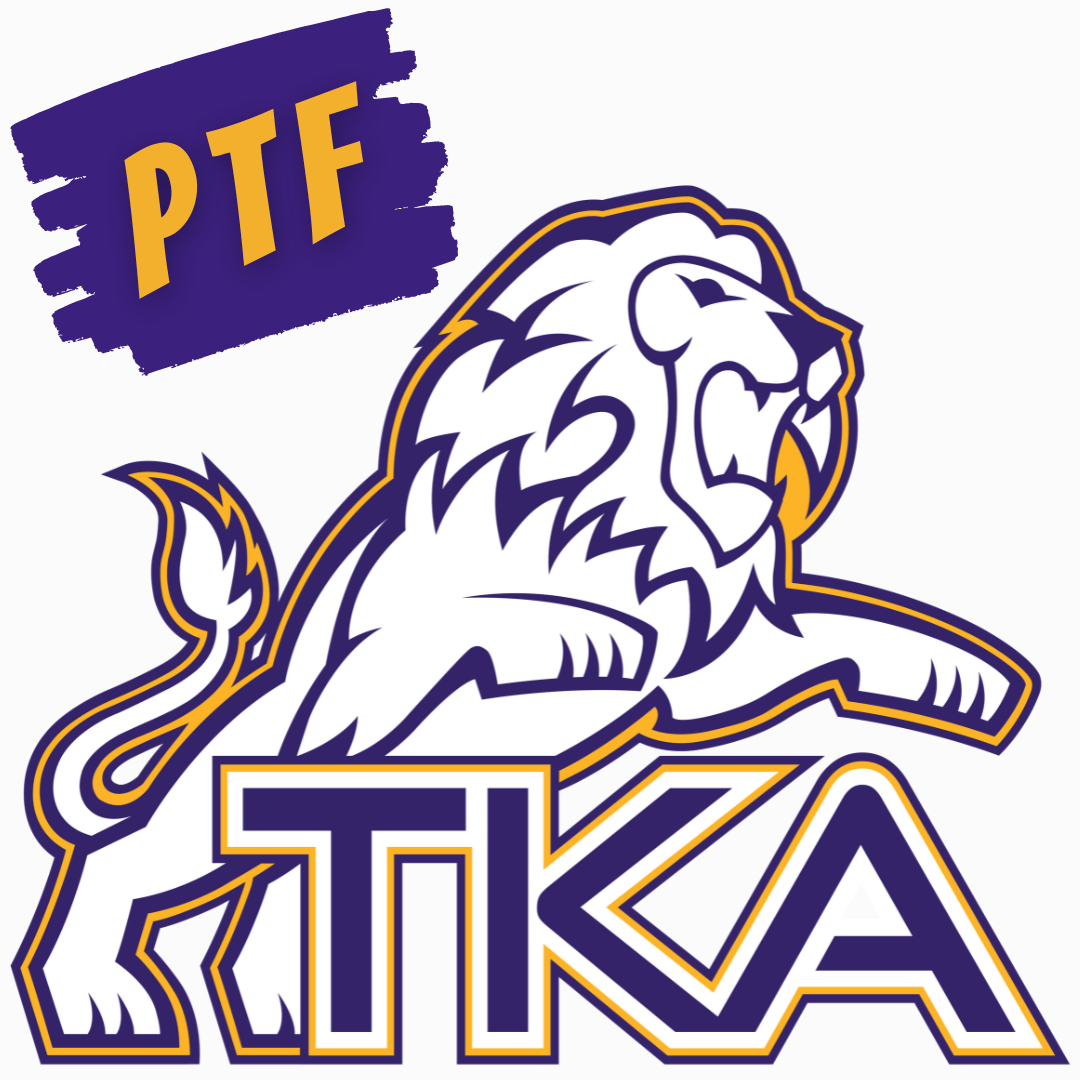# PTF Meeting

The first PTF meeting for this year will take place on Thursday, August 18, at 3:15 in Brandes.

Childcare will be provided through aftercare until 4:00 p.m. for those attending the meeting.

If you have not joined PTF for the 2022-23 school year, click this link below to sign up today!

 table div table+table+table+table+table+table+table+table+table+table+table+table+table+table+table+table+table div table{width:100%;padding:0}table div table+table+table+table+table+table+table+table+table+table+table+table+table+table+table+table+table div table img{width:96.23%;padding:0;float:none}table div table+table+table+table+table+table+table+table+table+table+table+table+table+table+table+table+table div table td{width:100%;padding:0 1.88% 18px}/* styles */# 2021-22 Yearbooks

Preordered yearbooks, are in and will be delivered to students over the next few days! If you did not order a yearbook for 2021-22, they can be purchased for \$55 in either school office or by emailing Mr. Josh Smith jsmith@thekingsacademy.net.

 table div table+table+table+table+table+table+table+table+table+table+table+table+table+table+table+table+table+table+table div table{width:100%;padding:0}table div table+table+table+table+table+table+table+table+table+table+table+table+table+table+table+table+table+table+table div table img{width:96.23%;padding:0;float:none}table div table+table+table+table+table+table+table+table+table+table+table+table+table+table+table+table+table+table+table div table td{width:100%;padding:0 1.88% 18px}/* styles */# The King's Harvesters Garden Club

Calling all students that love to get a little dirty and grow some amazing plants! The King's Harvesters Garden Club is ready to start up again Thursday, Aug. 18, from 3:15-4:15 pm every Thursday. The \$20 membership fee will be for the entire year. We are accepting up to 10 students.

Middle School Beta students and all High School students will receive community service hours. Middle School Beta students will be responsible for using the Beta App to log in individual hours and check in with Mrs. Brooks. All High School students will need to complete the community service form available on the FACTS Family Portal and turn it in to Mrs. Hatmaker.

If your Middle School or High School student would like to participate this year, parents will need to complete the following Google Form by Aug. 16.table div table+table+table+table+table+table+table+table+table+table+table+table+table+table+table+table+table+table+table+table+table+table+table div table{width:100%;padding:0}table div table+table+table+table+table+table+table+table+table+table+table+table+table+table+table+table+table+table+table+table+table+table+table div table img{width:96.23%;padding:0;float:none}table div table+table+table+table+table+table+table+table+table+table+table+table+table+table+table+table+table+table+table+table+table+table+table div table td{width:100%;padding:0 1.88% 18px}/* styles */# Varsity Volleyball

Our high school volleyball team is looking for more players! If you would like to play, contact Coach Danielle at dquesinberry@thekingsacademy.net.

 table div table+table+table+table+table+table+table+table+table+table+table+table+table+table+table+table+table+table+table+table+table+table+table+table+table div table{width:100%;padding:0}table div table+table+table+table+table+table+table+table+table+table+table+table+table+table+table+table+table+table+table+table+table+table+table+table+table div table img{width:96.23%;padding:0;float:none}table div table+table+table+table+table+table+table+table+table+table+table+table+table+table+table+table+table+table+table+table+table+table+table+table+table div table td{width:100%;padding:0 1.88% 18px}/* styles */# Sports Medicine Student Aid

The King's Academy Sports Medicine Program, under the guidance of Ortho Tennessee, is looking for a few dedicated student aids to help our athletic trainer with some of the day-to-day activities surrounding our sports teams. Interested students would need to have an interest in the healthcare field, be in good academic standing, and display a servant's heart as found in 1 Peter 4:10.

This fun and exciting opportunity will allow students to learn firsthand about sports first aid and CPR. Plus, they will have an opportunity to become part of the TKA Lions athletic culture.

Service learning hours may apply. Students would need to be available for 7th-period athletics and after-school practices, as well as some games.

 table div table+table+table+table+table+table+table+table+table+table+table+table+table+table+table+table+table+table+table+table+table+table+table+table+table+table+table div table{width:100%;padding:0}table div table+table+table+table+table+table+table+table+table+table+table+table+table+table+table+table+table+table+table+table+table+table+table+table+table+table+table div table img{width:96.23%;padding:0;float:none}table div table+table+table+table+table+table+table+table+table+table+table+table+table+table+table+table+table+table+table+table+table+table+table+table+table+table+table div table td{width:100%;padding:0 1.88% 18px}/* styles */# We're Hiring!

TKA has an employment opportunity available in our teaching faculty.

High School History Teacher TKA is seeking an employee to faithfully serve on our teaching faculty. Please email resumes to HR@thekingsacademy.net or call 865-573-8321.

 table div table+table+table+table+table+table+table+table+table+table+table+table+table+table+table+table+table+table+table+table+table+table+table+table+table+table+table+table+table div table{width:100%;padding:0}table div table+table+table+table+table+table+table+table+table+table+table+table+table+table+table+table+table+table+table+table+table+table+table+table+table+table+table+table+table div table img{width:96.23%;padding:0;float:none}table div table+table+table+table+table+table+table+table+table+table+table+table+table+table+table+table+table+table+table+table+table+table+table+table+table+table+table+table+table div table td{width:100%;padding:0 1.88% 18px}/* styles */# 6th - 8th Washington D.C. Trip

## March 6-9, 2023

How to register:
1. Go to: acts-tours.com
2. Enter Tour Code: T232036A

*You will be required to answer some questions, read and agree to terms, pay deposit, etc. Additional insurance can be purchased at this time.

 table div table+table+table+table+table+table+table+table+table+table+table+table+table+table+table+table+table+table+table+table+table+table+table+table+table+table+table+table+table+table+table div table{width:100%;padding:0}table div table+table+table+table+table+table+table+table+table+table+table+table+table+table+table+table+table+table+table+table+table+table+table+table+table+table+table+table+table+table+table div table img{width:96.23%;padding:0;float:none}table div table+table+table+table+table+table+table+table+table+table+table+table+table+table+table+table+table+table+table+table+table+table+table+table+table+table+table+table+table+table+table div table td{width:100%;padding:0 1.88% 18px}/* styles */# 9-12th Spring Break Trip: France, Germany and The Alps

## March 11-18, 2023

 table div table+table+table+table+table+table+table+table+table+table+table+table+table+table+table+table+table+table+table+table+table+table+table+table+table+table+table+table+table+table+table+table+table div table{width:100%;padding:0}table div table+table+table+table+table+table+table+table+table+table+table+table+table+table+table+table+table+table+table+table+table+table+table+table+table+table+table+table+table+table+table+table+table div table img{width:96.23%;padding:0;float:none}table div table+table+table+table+table+table+table+table+table+table+table+table+table+table+table+table+table+table+table+table+table+table+table+table+table+table+table+table+table+table+table+table+table div table td{width:100%;padding:0 1.88% 18px}/* styles */# TKA Athletic Banners

Do you have a business or know someone who does? TKA Athletics Banners are available for purchase now! These 3' by 5' banners will be proudly displayed in our Athletic facilities on campus for the 2022-2023 school year.

 table div table+table+table+table+table+table+table+table+table+table+table+table+table+table+table+table+table+table+table+table+table+table+table+table+table+table+table+table+table+table+table+table+table+table+table div table{width:100%;padding:0}table div table+table+table+table+table+table+table+table+table+table+table+table+table+table+table+table+table+table+table+table+table+table+table+table+table+table+table+table+table+table+table+table+table+table+table div table img{width:96.23%;padding:0;float:none}table div table+table+table+table+table+table+table+table+table+table+table+table+table+table+table+table+table+table+table+table+table+table+table+table+table+table+table+table+table+table+table+table+table+table+table div table td{width:100%;padding:0 1.88% 18px}/* styles */# TKA Garden and Greenhouse

More of our Lions participated in the Seymour Farmers Market with the TKA Garden and Greenhouse. They were selling the produce they started from seed last spring while participating in The King's Harvesters Garden Club! Most of the produce that was left over went straight to the TKA cafeteria to add to the daily incredible yummy lunches our school provides each week.

Come by and see what wonderful veggies we are growing at the TKA Garden!

The Seymour Farmers Market is each Saturday morning (8-12pm) at First Baptist Church of Seymour. We would love to see you there.

 table div table+table+table+table+table+table+table+table+table+table+table+table+table+table+table+table+table+table+table+table+table+table+table+table+table+table+table+table+table+table+table+table+table+table+table+table+table div table{width:100%;padding:0}table div table+table+table+table+table+table+table+table+table+table+table+table+table+table+table+table+table+table+table+table+table+table+table+table+table+table+table+table+table+table+table+table+table+table+table+table+table div table img{width:96.23%;padding:0;float:none}table div table+table+table+table+table+table+table+table+table+table+table+table+table+table+table+table+table+table+table+table+table+table+table+table+table+table+table+table+table+table+table+table+table+table+table+table+table div table td{width:100%;padding:0 1.88% 18px}/* styles */# Weekly Sports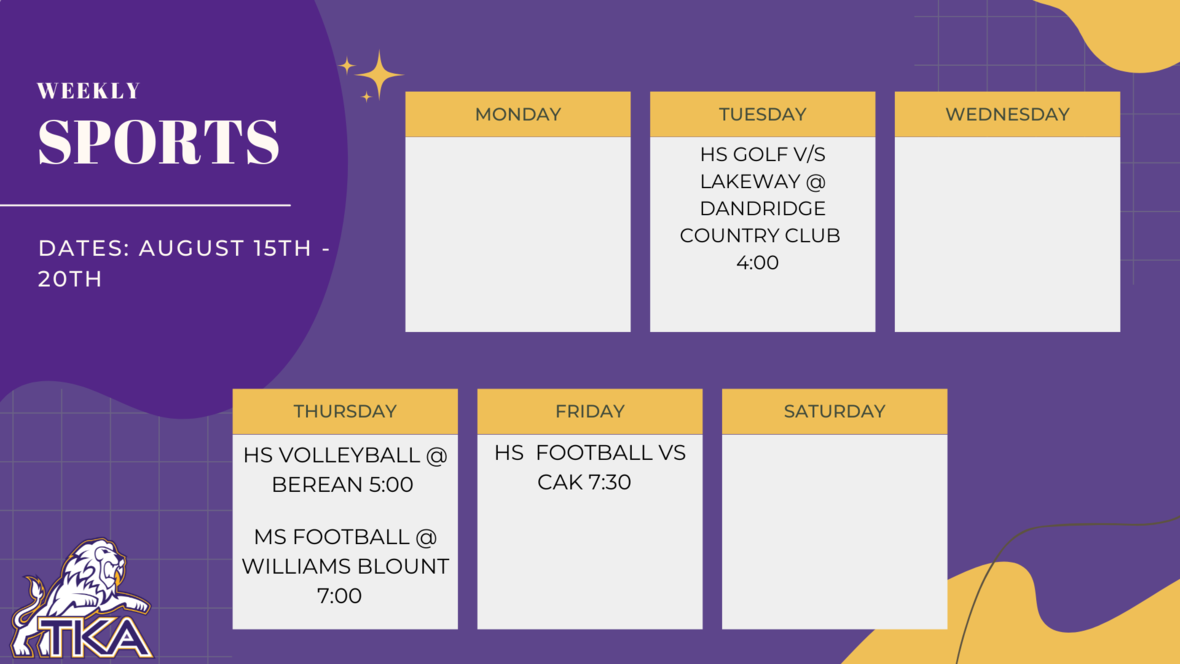table div table+table+table+table+table+table+table+table+table+table+table+table+table+table+table+table+table+table+table+table+table+table+table+table+table+table+table+table+table+table+table+table+table+table+table+table+table+table+table+table div table{width:100%;padding:0}table div table+table+table+table+table+table+table+table+table+table+table+table+table+table+table+table+table+table+table+table+table+table+table+table+table+table+table+table+table+table+table+table+table+table+table+table+table+table+table+table div table img{width:96.23%;padding:0;float:none}table div table+table+table+table+table+table+table+table+table+table+table+table+table+table+table+table+table+table+table+table+table+table+table+table+table+table+table+table+table+table+table+table+table+table+table+table+table+table+table+table div table td{width:100%;padding:0 1.88% 18px}/* styles */# Important information for the 2022-2023 School Year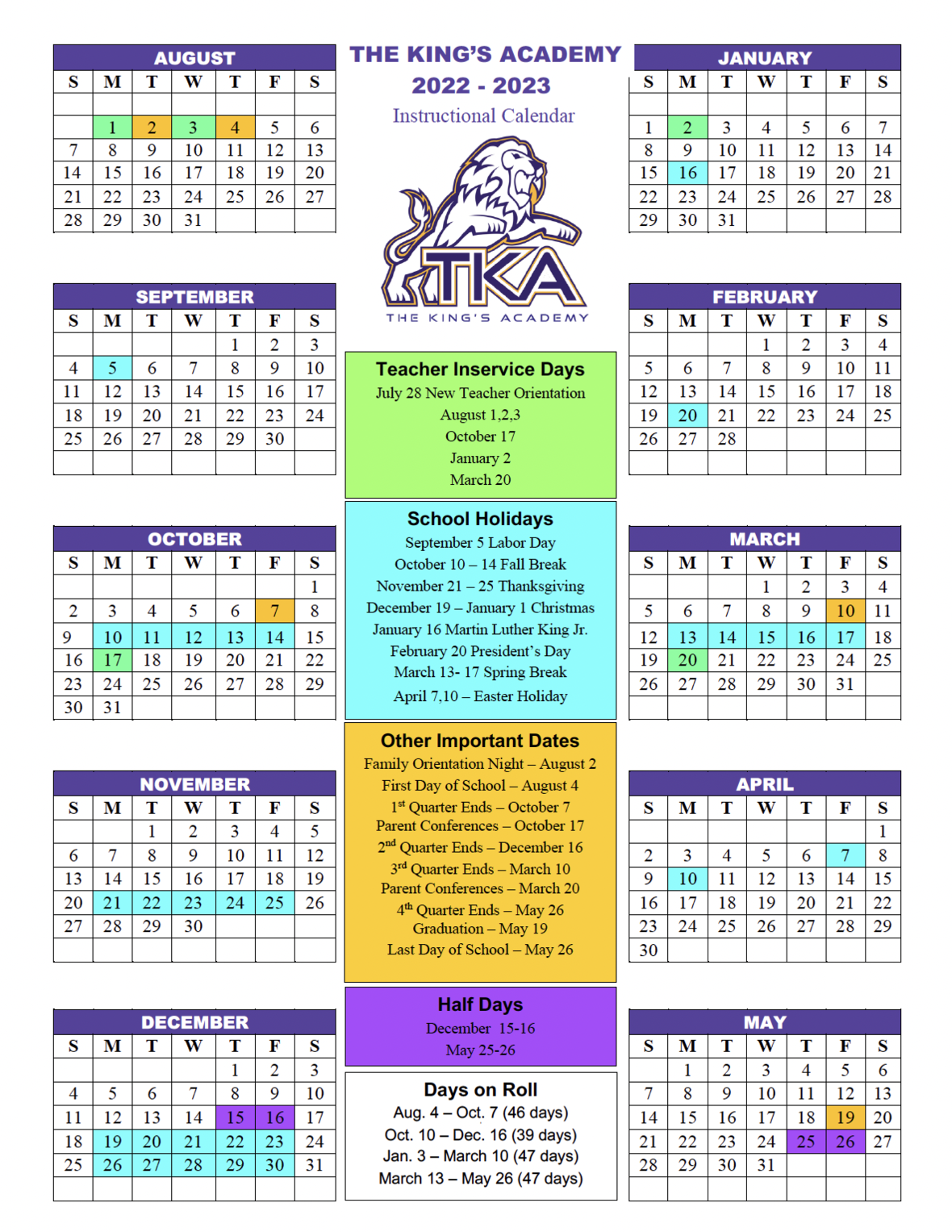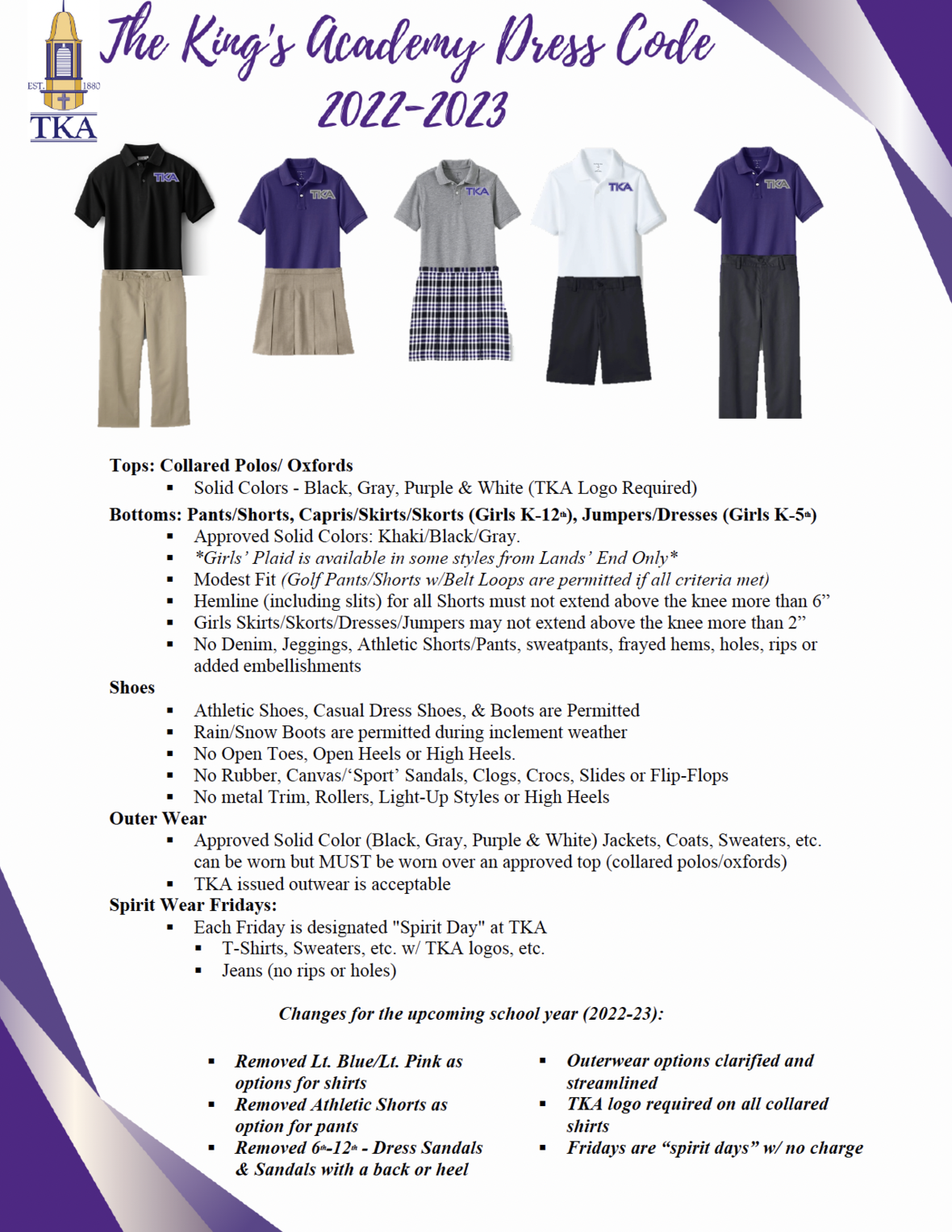table div table+table+table+table+table+table+table+table+table+table+table+table+table+table+table+table+table+table+table+table+table+table+table+table+table+table+table+table+table+table+table+table+table+table+table+table+table+table+table+table+table+table+table+table div table td,table.module-43{width:100%;padding:0}table div table+table+table+table+table+table+table+table+table+table+table+table+table+table+table+table+table+table+table+table+table+table+table+table+table+table+table+table+table+table+table+table+table+table+table+table+table+table+table+table+table+table+table+table div table{width:100%;float:none;margin-left:auto;margin-right:auto;padding:0}table div table+table+table+table+table+table+table+table+table+table+table+table+table+table+table+table+table+table+table+table+table+table+table+table+table+table+table+table+table+table+table+table+table+table+table+table+table+table+table+table+table+table+table+table div table a{border:0 none;text-decoration:none}table div table+table+table+table+table+table+table+table+table+table+table+table+table+table+table+table+table+table+table+table+table+table+table+table+table+table+table+table+table+table+table+table+table+table+table+table+table+table+table+table+table+table+table+table div table img{width:100%!important;border:0 none;text-decoration:none}/* styles */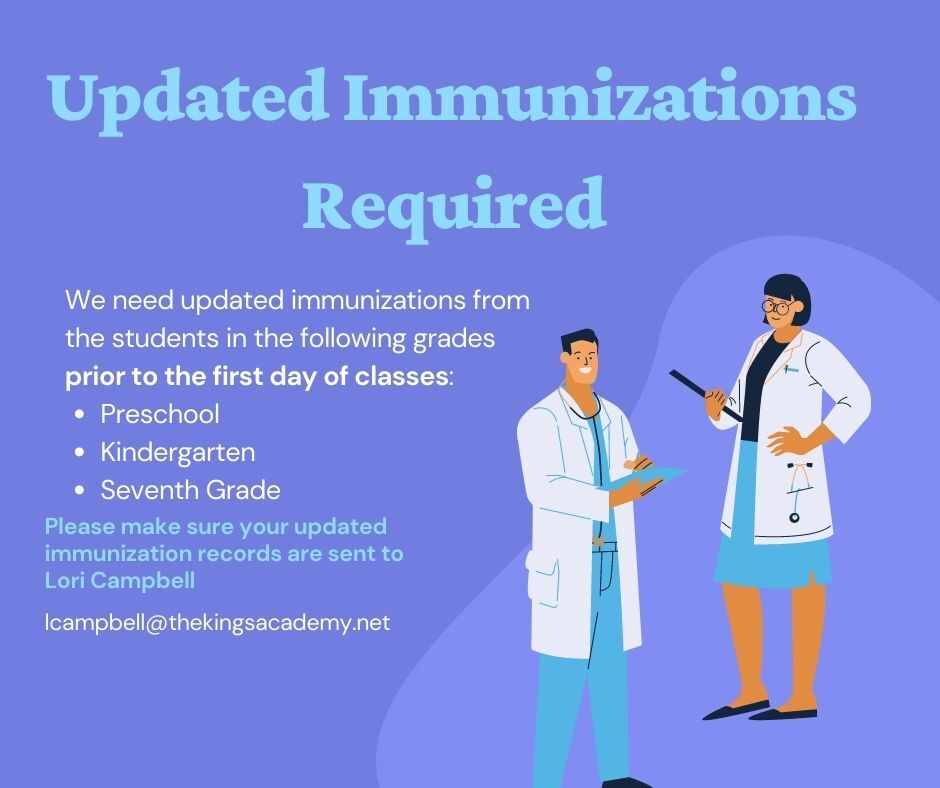table div table+table+table+table+table+table+table+table+table+table+table+table+table+table+table+table+table+table+table+table+table+table+table+table+table+table+table+table+table+table+table+table+table+table+table+table+table+table+table+table+table+table+table+table+table+table div table{width:100%;padding:0}table div table+table+table+table+table+table+table+table+table+table+table+table+table+table+table+table+table+table+table+table+table+table+table+table+table+table+table+table+table+table+table+table+table+table+table+table+table+table+table+table+table+table+table+table+table+table div table img{width:96.23%;padding:0;float:none}table div table+table+table+table+table+table+table+table+table+table+table+table+table+table+table+table+table+table+table+table+table+table+table+table+table+table+table+table+table+table+table+table+table+table+table+table+table+table+table+table+table+table+table+table+table+table div table td{width:100%;padding:0 1.88% 18px}/* styles */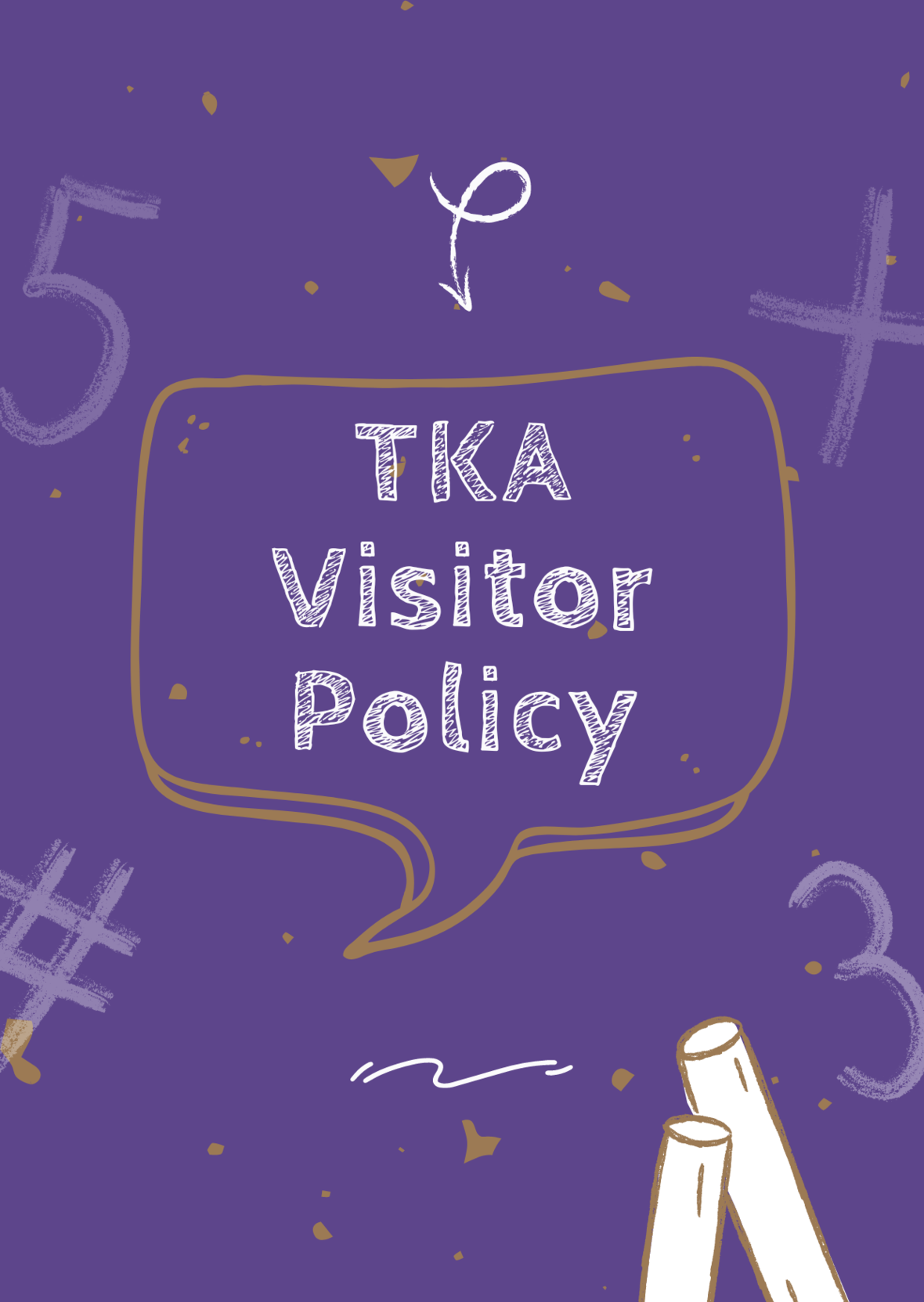# TKA Visitor Policy

In order to help maintain a safe and instructionally based learning environment for our students, all visitors for middle and high school must enter through the front door of the Anderson Building and sign in in the middle/high school office in order to receive a visitor's pass. All visitors for elementary must enter the front door of the Ogle Building in order to receive a visitor's pass. This pass should be visible at all times so that TKA faculty and staff know that you have checked in at the office.

Our doors are open to visitors, but please call ahead of time to schedule conferences with your child’s teachers. This will prevent interruptions to instruction and the loss of the valuable learning time of our students. Adults will not be allowed to wander the halls of the building or hold conferences with teachers during instructional times.

TKA family lunch visitors should follow the above procedure. Lunch tickets are \$5 each.

Students who are not enrolled at TKA are not permitted to visit TKA students during the school day, which includes lunch.

This policy has been developed in order to help maintain a safe and instruction focused environment at school each day. Any visitor who does not meet these expectations may be asked to leave school grounds.

 table div table+table+table+table+table+table+table+table+table+table+table+table+table+table+table+table+table+table+table+table+table+table+table+table+table+table+table+table+table+table+table+table+table+table+table+table+table+table+table+table+table+table+table+table+table+table+table+table div table{width:100%;padding:0}table div table+table+table+table+table+table+table+table+table+table+table+table+table+table+table+table+table+table+table+table+table+table+table+table+table+table+table+table+table+table+table+table+table+table+table+table+table+table+table+table+table+table+table+table+table+table+table+table div table img{width:96.23%;padding:0;float:none}table div table+table+table+table+table+table+table+table+table+table+table+table+table+table+table+table+table+table+table+table+table+table+table+table+table+table+table+table+table+table+table+table+table+table+table+table+table+table+table+table+table+table+table+table+table+table+table+table div table td{width:100%;padding:0 1.88% 18px}/* styles */# TKA Middle and High School Art:

To view the amazon wishlist for Middle and High school art, click here.

 table div table+table+table+table+table+table+table+table+table+table+table+table+table+table+table+table+table+table+table+table+table+table+table+table+table+table+table+table+table+table+table+table+table+table+table+table+table+table+table+table+table+table+table+table+table+table+table+table+table+table div table{width:100%;padding:0}table div table+table+table+table+table+table+table+table+table+table+table+table+table+table+table+table+table+table+table+table+table+table+table+table+table+table+table+table+table+table+table+table+table+table+table+table+table+table+table+table+table+table+table+table+table+table+table+table+table+table div table img{width:96.23%;padding:0;float:none}table div table+table+table+table+table+table+table+table+table+table+table+table+table+table+table+table+table+table+table+table+table+table+table+table+table+table+table+table+table+table+table+table+table+table+table+table+table+table+table+table+table+table+table+table+table+table+table+table+table+table div table td{width:100%;padding:0 1.88% 18px}/* styles */# 2022 - 2023 Elementary Clubs

TKA offers a variety of clubs to our Lion family. The Elementary Club list and fall schedule will be sent out in August. Keep an eye out for more information about these fun clubs!

 table div table+table+table+table+table+table+table+table+table+table+table+table+table+table+table+table+table+table+table+table+table+table+table+table+table+table+table+table+table+table+table+table+table+table+table+table+table+table+table+table+table+table+table+table+table+table+table+table+table+table+table+table div table{width:100%;padding:0}table div table+table+table+table+table+table+table+table+table+table+table+table+table+table+table+table+table+table+table+table+table+table+table+table+table+table+table+table+table+table+table+table+table+table+table+table+table+table+table+table+table+table+table+table+table+table+table+table+table+table+table+table div table img{width:96.23%;padding:0;float:none}table div table+table+table+table+table+table+table+table+table+table+table+table+table+table+table+table+table+table+table+table+table+table+table+table+table+table+table+table+table+table+table+table+table+table+table+table+table+table+table+table+table+table+table+table+table+table+table+table+table+table+table+table div table td{width:100%;padding:0 1.88% 18px}/* styles */TKA's football team will be selling 2022-2023 calendars. Save your advertising spot today!

Pricing for the Ads are as follows:

▪ \$750 Back Cover (Full Page)
▪ \$500 Full Page
▪ \$250 Half Page
▪ \$125 Quarter Page
▪ \$75 Side Page
 ▪ \$750 Back Cover (Full Page)
 ▪ \$500 Full Page
 ▪ \$250 Half Page
 ▪ \$125 Quarter Page
 ▪ \$75 Side Page

 table div table+table+table+table+table+table+table+table+table+table+table+table+table+table+table+table+table+table+table+table+table+table+table+table+table+table+table+table+table+table+table+table+table+table+table+table+table+table+table+table+table+table+table+table+table+table+table+table+table+table+table+table+table+table div table{width:100%;padding:0}table div table+table+table+table+table+table+table+table+table+table+table+table+table+table+table+table+table+table+table+table+table+table+table+table+table+table+table+table+table+table+table+table+table+table+table+table+table+table+table+table+table+table+table+table+table+table+table+table+table+table+table+table+table+table div table img{width:96.23%;padding:0;float:none}table div table+table+table+table+table+table+table+table+table+table+table+table+table+table+table+table+table+table+table+table+table+table+table+table+table+table+table+table+table+table+table+table+table+table+table+table+table+table+table+table+table+table+table+table+table+table+table+table+table+table+table+table+table+table div table td{width:100%;padding:0 1.88% 18px}/* styles */table div table+table+table+table+table+table+table+table+table+table+table+table+table+table+table+table+table+table+table+table+table+table+table+table+table+table+table+table+table+table+table+table+table+table+table+table+table+table+table+table+table+table+table+table+table+table+table+table+table+table+table+table+table+table+table+table+table div table{width:100%;padding:0}table div table+table+table+table+table+table+table+table+table+table+table+table+table+table+table+table+table+table+table+table+table+table+table+table+table+table+table+table+table+table+table+table+table+table+table+table+table+table+table+table+table+table+table+table+table+table+table+table+table+table+table+table+table+table+table+table+table div table img{width:96.23%;padding:0;float:none}table div table+table+table+table+table+table+table+table+table+table+table+table+table+table+table+table+table+table+table+table+table+table+table+table+table+table+table+table+table+table+table+table+table+table+table+table+table+table+table+table+table+table+table+table+table+table+table+table+table+table+table+table+table+table+table+table+table div table td{width:100%;padding:0 1.88% 18px}/* styles */table div table+table+table+table+table+table+table+table+table+table+table+table+table+table+table+table+table+table+table+table+table+table+table+table+table+table+table+table+table+table+table+table+table+table+table+table+table+table+table+table+table+table+table+table+table+table+table+table+table+table+table+table+table+table+table+table+table+table+table div table{width:100%;padding:0}table div table+table+table+table+table+table+table+table+table+table+table+table+table+table+table+table+table+table+table+table+table+table+table+table+table+table+table+table+table+table+table+table+table+table+table+table+table+table+table+table+table+table+table+table+table+table+table+table+table+table+table+table+table+table+table+table+table+table+table div table img{width:96.23%;padding:0;float:none}table div table+table+table+table+table+table+table+table+table+table+table+table+table+table+table+table+table+table+table+table+table+table+table+table+table+table+table+table+table+table+table+table+table+table+table+table+table+table+table+table+table+table+table+table+table+table+table+table+table+table+table+table+table+table+table+table+table+table+table div table td{width:100%;padding:0 1.88% 18px}/* styles */